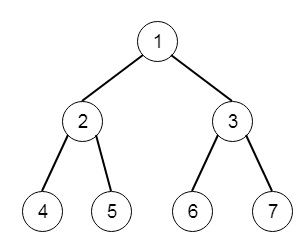# Construct Binary Tree from Preorder and Postorder Traversal in Python

Suppose we have two traversal sequences Preorder and Postorder, we have to generate the binary tree from these two sequences. So if the sequences are [1,2,4,5,3,6,7], [4,5,2,6,7,3,1], then the output will beTo solve this, we will follow these steps −

• ans := make a tree node by taking value pre, stack := empty stack, and insert ans
• i := 1 and j := 0
• while i < length of pre and j < length of post
• if stack top value = post[j], then increase j by 1, pop from stack, and go for next iteration
• node := make a tree node with value pre[i]
• if left part of stack top node is empty, then set left of stack top node as node, otherwise set right of stack top node as node
• insert node into stack
• increase i by 1
• return ans

Let us see the following implementation to get better understanding −

## Example

class TreeNode:
def __init__(self, data, left = None, right = None):
self.data = data
self.left = left
self.right = right
def height(root):
if root is None:
return 0
else :
# Compute the height of left and right subtree
l_height = height(root.left)
r_height = height(root.right)
#Find the greater one, and return it
if l_height > r_height :
return l_height+1
else:
return r_height+1
def print_given_level(root, level):
if root is None:
return
if level == 1:
print(root.data,end = ',')
elif level > 1 :
print_given_level(root.left , level-1)
print_given_level(root.right , level-1)
def level_order(root):
print('[', end = '')
h = height(root)
for i in range(1, h+1):
print_given_level(root, i)
print(']')
class Solution(object):
def constructFromPrePost(self, pre, post):
"""
:type pre: List[int]
:type post: List[int]
:rtype: TreeNode
"""
ans = TreeNode(pre)
stack = [ans]
i = 1
j = 0
while i < len(pre) and j < len(post):
if stack[-1].data == post[j]:
j+=1
stack.pop(-1)
continue
node = TreeNode(pre[i])
if not stack[-1].left:
stack[-1].left = node
else:
stack[-1].right = node
stack.append(node)
i+=1
return ans
ob = Solution()
pre = [1,2,4,5,3,6,7]
post = [4,5,2,6,7,3,1]
tree = ob.constructFromPrePost(pre, post)
level_order(tree)

## Input

[1,2,4,5,3,6,7]
[4,5,2,6,7,3,1]
pre = [1,2,4,5,3,6,7]
post = [4,5,2,6,7,3,1]

## Output

[1,2,3,4,5,6,7,]